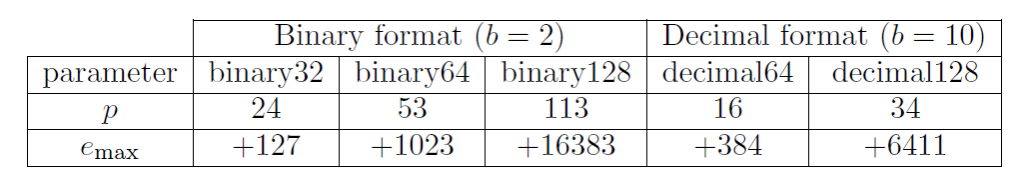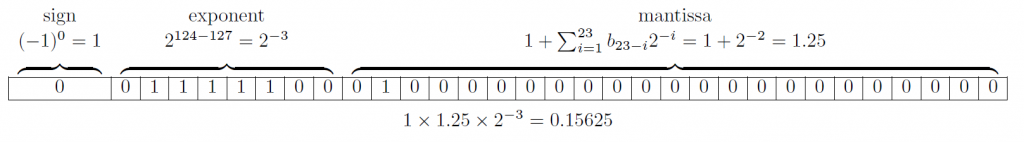# Real number approximations in finite space with IEEE 754

#### Jeffrey Quesnelle

Computer systems often need to represent real numbers as a part of their operation, but how to encode these numbers in fixed, finite space is non-trivial. If the size of the variable in question is bounded then so-called fixed point arithmetic can be used, treating both sides of the decimal point as integer values. In general however it would be useful to have a more flexible method of representing values that can hold both $10$ and $10^{-9}$ in a small, fixed amount of memory.

The current base ten number system is a relatively new invention . We may take for granted that there is a well-defined way of writing down numbers (with only terminating reals having non-unique representations) but the problem of representing an arbitrary real number in fixed space (say, in computer memory) raises several interesting tradeoffs between precision and accuracy; we give an extremely abbreviated overview of the most popular method of representing reals in computers: IEEE 754.

IEEE Standard for Floating-Point Arithmetic (IEEE 754-2008 or simply 754) is the internationally accepted method for performing operations on and transmitting approximations of reals on computer systems. The key property of 754 is that the decimal point “floats”, i.e. if a number is very large or very small the decimal point can be “moved around” so that most bits are used to represent the significant digits of the number. Compare this method to fixed point arithmetic which has a bias towards numbers closer to zero; in 8.8 fixed point math (8 bits for whole part, 8 bits for fractional part) the number $2^{7}$ is represented as $1000 \; 0000.0000 \; 0000$ and $2^8$ cannot be represented at all, even though both numbers contain only one “significant digit”!

Every IEEE 754-2008 number is of the form: $(-1)^s \times b^q \times c$ where $s$ (the sign) is 0 or 1, $b$ is 2 or 10 (the base), $q \in \mathbb Z$ with $e_{\text{min}} \le q+p-1 \le e_{\text{max}}$ (the exponent), and $c \in \mathbb N$ with $0 \le c \le b^p$ (the significand, coefficient or mantissa) . IEEE 754-2008 defines several formats, which are values for $p$ (the digits) and $e_{\text{max}}$ (we always have that $e_{\text{min}} = -e_{\text{max}} + 1$). These formats are given in Figure 1. In addition to the finite numbers, each format also has four special “numbers”: two infinites ($+\infty$ and $-\infty$) and two NaNs or not a number (qNaN and sNaN) which represent special states and error conditions.Figure 1: Parameters of basic formats in IEEE 754-2008

Using an exponent and mantissa representation gives rise to the floating point nature of IEEE 754. For example, the number $-12.345$ is represented in decimal64 as $s=1, c=12345, e=-3$. Likewise $1,234.5$ is represented as $s=0, c=12345, e=-1$. To encode these values in computer memory each parameter is given a fixed number of bits based on the format. For example the encoding (or binary interchange format) of $0.15625$ in binary32 is shown in Figure 2.Figure 2: Example of binary32 (or single-precision float) encoding

Computer systems that use IEEE 754-2008 must be aware of its idiosyncrasies. By perhaps the most obtuse use of the pigeon hole principle ever, not all real numbers can be represented in finite space. Because computers almost exclusively use the binary formats, even simple base ten arithmetic may produce unexpected results. For example, consider the $b^2-4ac$ radicand in the quadratic formula: if $b=3.34, a =1.22, c=2.28$ then $b^2-4ac = 0.292$, but with single-precision IEEE 754 arithmetic the result is $0.0291996$. This may be further complicated by the specific behavior of the compiler — in general, floating point numbers should not be used when result must be computed exactly.

 Katz, Victor J. A History of Mathematics: An Introduction. Boston: Addison-Wesley, 2009.
 IEEE Standard for Floating-Point Arithmetic, IEEE Standard 754, 2008.